## LetsPlayMaths.Com

WELCOME TO THE WORLD OF MATHEMATICS

# Class 3 Addition

Class 2 Recap

Addition Without Carrying

Addition With Carrying

Story Time Addition

Addition Test

Addition Worksheet

Answer Sheet

## Class 2 Recap

In Standard two, we learnt how to add 3-digits numbers with or without carrying. Few examples are shown below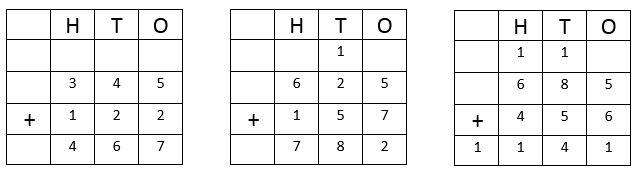## Addition Without Carrying

The same 3-digit numbers addition method is applied to 4-digits, 5-digits and 6-digits. Let us learn 4-digits
or 5-digits addition without carrying. Few examples are given below.

Example 1. Add 5345 and 4122

Solution. Putting the numbers in tabular format as shown below.So, Sum of 5345 and 4122 is 9467

Example 2. Write in tabular format and add: 34635, 50231 and 3100.

Solution. Putting the numbers in the column form## Addition With Carrying

We have already learnt the addition on 2 and 3 digit numbers. We will use the same method for addition 4 and 5 digit numbers.

Example 1. Find the sum of 4745 and 4387.

Solution. Putting the numbers in column format as shown below.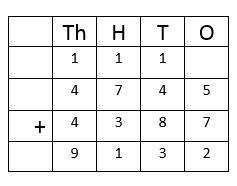Step 1: Add the ones place value numbers. 5 + 7 = 12 Ones = 1 Ten + 2 ones.

Write 2 under ones column and carry 1 ten to the tens place column.

Step 2: Add the tens. 8 + 4 + 1 (Carryover) = 13 Tens = 1 hundred + 3 tens

Write 3 under tens column and carry 1 hundred to the hundreds column.

Step 3: Add the hundreds. 7 + 3 + 1 (Carryover) = 11 hundreds = 1 Thousand + 1 hundred

Write 1 under hundreds column and carry 1 thousands to thousands column.

Step 4: Add the Thousands. 4 + 4 + 1 (Carryover) = 9 thousands

So, the answer is 9132.

Example 2. Find the sum of 15625, 26472 and 37928

Solution. Putting the numbers in column format as shown below.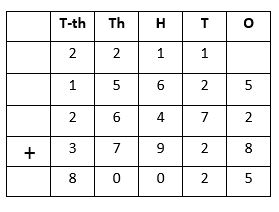So, the answer is 80025.

## Story Time Addition

In our day to day life we use concept of addition in many ways, few examples are shown below.

Example 1. A salesman sold \$ 4567 on Monday and on Tuesday he sold \$ 3928. Find out how much he sold on these days?

Solution.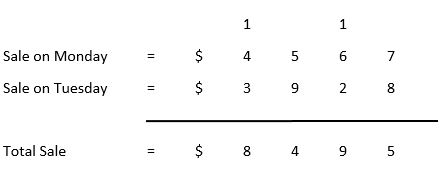So, the total sale is \$ 8495.

Example 1.Population of Philadelphia state is 89765 and population of Ohio state is 67569. What is the total population of both the sates?

Solution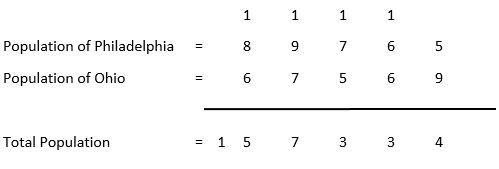So, the total population of both the cities are 157334.

## Addition Test

Addition Test - 1

Addition Test - 2

## Addition Worksheet

Addition Worksheet - 1

Addition Worksheet - 2

Addition Worksheet - 3

## Answer Sheet

Addition-AnswerDownload the pdf

Copyright © 2021 LetsPlayMaths.com. All Rights Reserved.